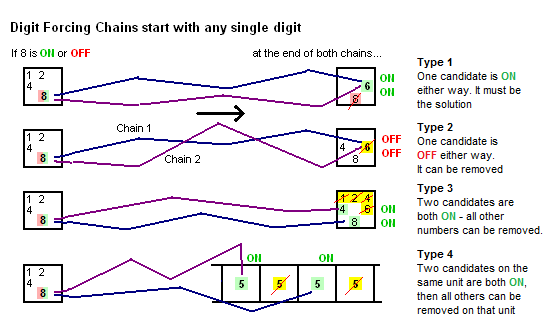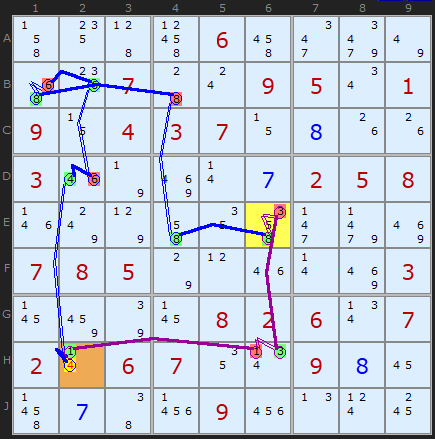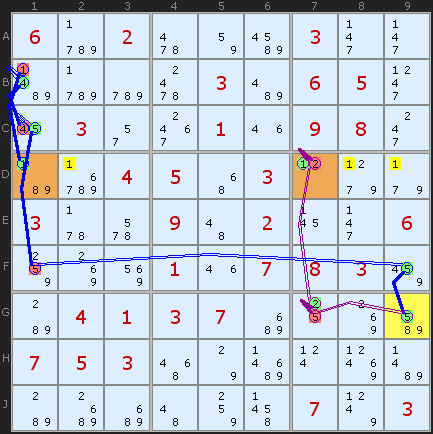Main Page - Back

# Digit Forcing Chains

From sudokuwiki.org, the puzzle solver's siteThis is the start of a family of strategies called Forcing Chains. As the name implies they are made from chains - or formally - Alternating Inference Chains which are simple ON - OFF- ON - OFF consequences. Chains can start with an ON or an OFF. When a candidate X it turned 'on' it immediately turns 'off' all other candidates it can see. When a candidate is in the 'off' state it might turn on another candidate if there is only one left in the unit it can see.

In the strategy Forcing Chains we look at the consequences of a candidate being first ON and then OFF, or a group of candidates in a cell being ON (Cell Forcing Chains) or a number being ON in all instances in a unit (Unit Forcing Chains). If the consequences of the chains are identical - that another candidate is always ON or always OFF - then we have a contradiction.Family of Digit Forcing Chains Before we look at specific examples, its worth going over the different types of attack in this family. In this diagram the starting cell is on the left - and an 8 is chosen. It is attacking either another digit (first two rows), a cell (middle two rows) or a unit (last two rows). In each attack the ends of the two chains are either ON or OFF.

If the two ends of the chain meet on the same digit, the we can remove that digit if the ends are OFF, or it's the solution to that cell if the chain ends are both ON.

Likewise, we can attack a whole cell by finding two ON or OFF digits. If OFF, then the last remaining candidate is the solution - but this only works if there are 3 candidates in the whole cell. If ON, then we know that one of those two ON cells is the solution, so any other candidates can be removed.

And finally, the unit attack, based on the same number appearing three or more times in that unit. You will see the pattern now. If we can find two numbers that the chain ends say must be ON, then one of them is the solution and the rest of that number X can be removed from the unit. If there are three numbers left and we identify two numbers that are off, then the last candidate is the solution.Figure 1: Digit Forcing Chain : Load Example or : From the Start In this article we have a very tough Sudoku which contains a neat series of Digit Forcing Chains showing several different consequences. The first is given in Figure 1.

The 'digit' in this strategy is a single candidate - in this case the 5 in J9. We are looking at the consequences of this 5 being ON or OFF. Following the blue chain where 5 is OFF we get to the cell E1 where the consequence of 5 being OFF is to turn 6 ON. This chain is (the blue chain):
-5[J9]+5[H9]-5[H5]+5[E5]-5[E4]+8[E4]
-8[B4]+8[B1]-6[B1]+6[E1]

Now, if the 5 in J9 were ON we can trace another shorter chain to E9 were we also find the a 6 can be turned ON. The chain for this is (the purple chain):
+5[J9]-2[J9]+2[C9]-6[C9]+6[E9]
So whether J9 is 5 or not, we know 6 will appear in row E in E1 or E9, so the other 6s in E2 and E8 can be removed.
In the very next step we are obliged to find another Digit Forcing Chain this time centered on 8 in E4. Like the first example it finds that either way the 8 in E2 will give us a 5 either on H5 (the blue chain) or a 5 on H9. This either/or situation establishes 5 in one of those two cells so the remaining 5 in H2 can be removed.
The blue chain is:
-8[E4]+5[E4]-5[E5]+5[H5]
The purple chain +8[E4]-8[B4]+8[B1]-6[B1]+6[E1]
-6[E9]+4{E9|A9}-4[H9]+5[H9]
contains an awkward ALS on
{E9|A9} - not easy to spot. The chain goes into the cell E9 and turns OFF the 6 which leaves just a Naked Pair of 4/9 in A9 and H9. That in turn removes the 4 in H9 giving us the second fixed 5 in H9.

## Digit to Unit attackFigure 3: Third Digit Forcing Chain Lastly in this Sudoku we find one of the more common types of Digit Forcing Chain, one that fixes a digit in another cell. E6 is the start cell 8 is the digit we are flipping ON or OFF. If OFF we take the blue chain around the board to H2 where 1 gets turned ON. This chain is:
-8[E6]+8[E4]-8[B4]+8[B1]-6[B1]
+6[B2]-6[D2]+4[D2]-4[H2]+1[H2]

The consequence of 8 in E6 being ON also shows that 1 in H2 must be ON as well. The second, purple chain is +8[E6]-3[E6]+3[H6]-1[H6]+1[H2].
So, whether E6 is 8 or not the chains imply H2 must be 1 and that opens up the puzzle to the end game.

## Second ExampleDigit Forcing Chains aim at a Unit : Load Example or : From the Start Careful traversing of two chains might result in the chain ends resting on the same digit in the same row, column or box. The example here starts on G9 and winds it's way round to the 1s on row D. If the 5 in G9 is ON we get a result of 1 on D7. If the 5 in G9 is OFF we get a 1 on D1. Therefore, there cannot be any other 1s on that row. Three other 1s eliminated is a bit hit at this stage of the puzzle. The solver will return:

DIGIT FORCING CHAIN: because of G9, 1s in Row 4 are fixed on [D1,D7]
-5[G9]+5[F9]-5[F1]+5[C1]
-4[C1]+4[B1]-1[B1]+1[D1]
+5[G9]-5[G7]+2[G7]-2[D7]+1[D7]
we can remove 1 from D2
we can remove 1 from D8
we can remove 1 from D9